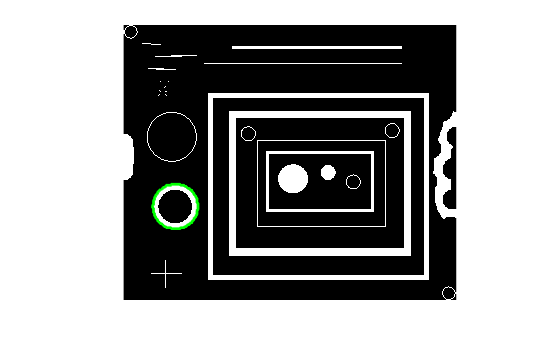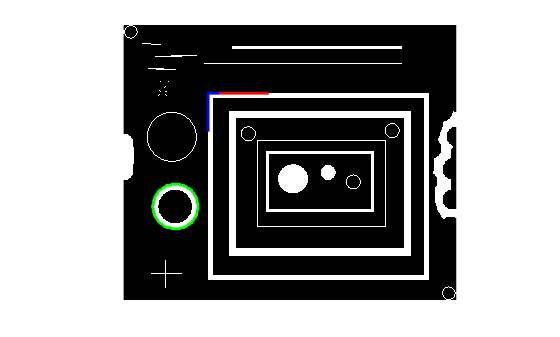Main Content

# bwtraceboundary

Trace object in binary image

## Syntax

``B = bwtraceboundary(BW,P,fstep)``
``B = bwtraceboundary(BW,P,fstep,conn)``
``B = bwtraceboundary(BW,P,fstep,conn,m,dir)``

## Description

````B = bwtraceboundary(BW,P,fstep)` traces the outline of an object in binary image `BW`. Nonzero pixels belong to an object and zero-valued pixels constitute the background. `P` specifies the row and column coordinates of the point on the object boundary where you want the tracing to begin. `fstep` specifies the initial search direction for the next object pixel connected to `P`. `B` holds the row and column coordinates of the boundary pixels for the region.```
````B = bwtraceboundary(BW,P,fstep,conn)` traces the boundary, where `conn` specifies the desired connectivity.```

example

````B = bwtraceboundary(BW,P,fstep,conn,m,dir)` specifies `m`, the maximum number of boundary pixels to extract, and `dir`, the direction in which to trace the boundary. By default, `bwtraceboundary` identifies all the pixels on the boundary.```

## Examples

collapse all

Read an image and display it.

```BW = imread('blobs.png'); imshow(BW)```Pick an object in the image and trace the boundary. To select an object, specify a pixel on its boundary. This example uses the coordinates of a pixel on the boundary of the thick white circle, obtained through visual inspection using `impixelinfo`. By default, `bwtraceboundary` identifies all pixels on the boundary.

```r1 = 163; c1 = 37; contour = bwtraceboundary(BW,[r1 c1],'W');```

Plot the contour on the image.

```hold on plot(contour(:,2),contour(:,1),'g','LineWidth',2)```Pick a point on the boundary of a second object. This example uses the coordinates of a pixel near the upper-left corner of the largest rectangle. Trace the first fifty boundary pixels in the clockwise direction.

```r2 = 68; c2 = 95; contourCW = bwtraceboundary(BW,[r2 c2],'W',8,50,'clockwise');```

Starting at the same point on the second object boundary, trace the first fifty boundary pixels in the counterclockwise direction.

`contourCCW = bwtraceboundary(BW,[r2 c2],'W',8,50,'counterclockwise');`

Plot the clockwise contour on the image in red. Plot the counterclockwise contour on the image in blue.

```plot(contourCW(:,2),contourCW(:,1),'r','LineWidth',2) plot(contourCCW(:,2),contourCCW(:,1),'b','LineWidth',2)```## Input Arguments

collapse all

Binary image, specified as a 2-D numeric or logical matrix.

Data Types: `single` | `double` | `int8` | `int16` | `int32` | `int64` | `uint8` | `uint16` | `uint32` | `uint64` | `logical`

Coordinates of starting point on the object boundary where you want the tracing to begin, specified as a 2-element vector of the format `[row column]`.

Data Types: `double`

Initial search direction for the next object pixel connected to `P`, specified as a character vector or string scalar as depicted in the diagram.Note

When the connectivity `conn` is `4`, `fstep` is limited to the values `'N'`, `'E'`, `'S'`, and `'W'`.

Data Types: `char` | `string`

Pixel connectivity, specified as `8` or `4`.

Value

Meaning

Two-Dimensional Connectivities

4-connected

Pixels are connected if their edges touch. The neighborhood of a pixel are the adjacent pixels in the horizontal or vertical direction.8-connected

Pixels are connected if their edges or corners touch. The neighborhood of a pixel are the adjacent pixels in the horizontal, vertical, or diagonal direction.Data Types: `double`

Maximum number of boundary pixels to extract, specified as a positive integer. By default, `m` is `Inf` and `bwtraceboundary` identifies all the pixels on the boundary.

Data Types: `double`

Direction in which to trace boundary, specified as `'clockwise'` or `'counterclockwise'`.

Data Types: `char` | `string`

## Output Arguments

collapse all

Row and column coordinates of the boundary pixels for the region, returned as a q-by-2 matrix. Each row in `B` has the form `[row column]`.

## Algorithms

The `bwtraceboundary` function implements the Moore-Neighbor tracing algorithm modified by Jacob's stopping criteria. This function is based on the `boundaries` function presented in the first edition of Digital Image Processing Using MATLAB, by Gonzalez, R. C., R. E. Woods, and S. L. Eddins, New Jersey, Pearson Prentice Hall, 2004.

 Gonzalez, R. C., R. E. Woods, and S. L. Eddins, Digital Image Processing Using MATLAB, New Jersey, Pearson Prentice Hall, 2004.

Download ebook